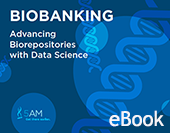# Up at 5AM: The 5AM Solutions Blog

This is the first in a series of blog posts that will dive more deeply into the nuts and bolts of Data Science. Today we will talk a bit about statistics, but we will be talking tools, visualization strategies, and data representation in the context of specific problems.

Let's say that you have a large database with millions of records. These records have wildly different provenance: some have been carefully curated by domain experts based on the best experimental results, and others are collected from lots and lots of unreliable experiments. The problem is that we have a lot of unreliable data, and not enough carefully curated data. For this exercise, let's assume that there is actual value in these unreliable experiments, just not a lot.

This is the central challenge of Big Data in science: how do we take potentially uncontrolled data and use it in a controlled experiment? We won't be solving that entire problem today, but this is one potential strategy that can be used. When we have a database of facts of varying quality, what we need to do is find a way to assess that quality. I like to use probability: that is, the probability that a given statement is true. In principle, the probability that an assertion is true is based on its provenance: what's the justification for that fact? Did someone we trust say it? Was a particular experimental method used to determine it? Here are a couple of simple (hence stupid) tricks that we can do with z-scores that can help us gain the insight we need into large, unreliable datasets.

## Nanopublications, Confidence, and Probability

We can use reasoning strategies to infer the probability that an assertion is true. For instance, I have used the Nanopublication framework with PROV-based provenance to say that "the proteins SLC4A8 is the target of CA2 in a direct interaction that was determined using a pull down experiment. Further, this was pulled from BioGRID and was published in the article "Regulation of the human NBC3 Na+/HCO3- cotransporter by carbonic anhydrase II and PKA".Given this information, we can now make some inferences, based on what we know about pull down experiments:

#### SubClassOf: Confidence2

That is, we can say that we have a confidence level of 2 on statements based on pull down experiments. But what does it mean to have a confidence level of 2 in a nanopublication? We can define what that means, by assigning it a probability:

#### SubClassOf: 'probability value' value 0.97

Where did we get the probability of 0.97 from? We made it up, based on our intuitions of what confidence scores of 0, 0.5, 1, 2, and 3 might be. I'm interpreting these scores as meaning "no evidence", "little evidence", "some evidence", "good evidence", and "great evidence" respectively. As it turns out, we can look to z-scores to formalize our intuitions. Z-scores are a way of "stretching out" probabilities as they approach zero and one. Below is the Cumulative Distribution Function (CDF) that shows the relationship between z-scores and probabilities, when data is normalized with a mean of 0 and a standard deviation of 1. Z-scores are also used to normalize differential gene expression data, and the transformation to probability can be interpreted as the probability that a gene is expressed in a given diseased sample but not in a normal sample.If we look at z-scores of 0, 1, 2, and 3, we can see that they map to the following probabilities:

 Evidence Level Z-Score Probability no evidence 0 0.5 little evidence 0.5 0.69 some evidence 1 0.84 good evidence 2 0.977 great evidence 3 0.997

This can be a good intuitive guide for confidence, since it's easier to think about these sorts of integers than trying to figure out how many 9's to tack on the end of a probability, while also providing a symmetric measure for how unlikely something is, which is essentially negation.## Experimental Replication

That's only the first trick that z-scores can provide. When we perform independent experiments with given quality and levels of confidence, we should be able to compute a consensus probability based on the fact that two or more experiments came to the same conclusion, just with different levels of support. Composite z-scores can help us compute that consensus. At it's heart, the sum of z-scores produces a composite z-score. However, to perform this calculation on probabilities, we have to apply the Cumulative Distribution Function (below, shown as F(x)) and its inverse to take a set of probabilities, turn them into z-scores, sum them, and turn that composite back into a probability:So using the intuitions laid out above, we can tell that if six experiments that show little evidence all agree, then the probability of error has been reduced, which means that it has the same composite probability as an experiment with great evidence. Additionally, if an experiment produces a negative result, it reduces the overall probability of an assertion being true. This models the heuristic that "extraordinary claims require extraordinary evidence". Finally, bare assertions with no evidence have no effect on the overall probability, since the z-score is zero.

These techniques allow us to adjust the confidence scores as-needed when we learn more about the kinds of evidence that have been gained. It also means that we can take advantage of weak and contradictory evidence in large heterogeneous datasets aggregated from many sources. I will be presenting these techniques today in a poster at the Conference on Semantics and Healthcare and Life Sciences. Please stop by if you have any questions.## Diagnostic Tests on the Map of BiomedicineDownload the ebook based on our popular blog series. This free, 50+ page edition features updated, expanded posts and redesigned, easier-to-read maps.

### FREE Biobanking EbookGet this 29 page PDF document on how data science can be used to advance biorepositories.

### Free NGS WhitepaperLearn about the applications, opportunities and challenges in this updated free white paper.